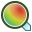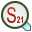# S-Parameter Goal

Optimise the S-parameters that are solved as part of the Feko model.

On the Request tab, in the Optimisation group, click theAdd goal function icon. From the drop down list, selectS-matrix goal.

The Focus is identified based on the label of a Multiport S-parameter request in CADFEKO or of an SP card in EDITFEKO.

## Quantity

Coupling coefficient (Smn)
Only the coupling between different ports will be considered in the optimisation (all S-parameter values where the port indices are not equal).
Note: If Snm and Smn are computed in an S-parameter request, then both of these values will be considered in the goal evaluation. If the coupling in one direction is required, the relevant port should be deactivated in the S-parameter calculation request (CADFEKO) or the source set to zero magnitude (EDITFEKO).
Reflection coefficient (Snn)
Only the reflection at the port(s) will be considered in the optimisation. The reflection coefficient at all ports that are active for the S-parameter computation will be considered.
Return loss
The return loss at the port(s) will be considered in the optimisation. Return loss is calculated from the reflection coefficient at each active port as:
(1) $\text{RL}=-20\mathrm{log}|{S}_{nn}|$
Transmission coefficient
The transmission coefficient at the port(s) will be considered in the optimisation. The transmission coefficient is calculated from:
(2) $\Upsilon =1-|{S}_{nn}|$
VSWR
The voltage standing wave ratio at the port(s) will be considered in the optimisation. Return loss is calculated from the reflection coefficient at each active port as:
(3) $\text{VSWR}=\frac{1+|{\text{S}}_{nn}|}{1-|{\text{S}}_{nn}|}$

## Port Selection

Specify input port number (n)
By default all of the active ports will be considered during the goal evaluation. When activated, this option allows the selection of a single port to be used as the input port. For example, if all of the S-parameters in a 3-port device are computed and the Focus quantity is chosen as Coupling coefficient (Smn), then if the input port is specified as 2, only the values of S12 and S32 will be considered during the goal evaluation.
Specify output port number (m):
In a similar manner to the input port selection option, when this option is selected it allows the selection of a single port to be used as the output port. For example, if all of the S-parameters in a 3-port are computed and the Focus quantity is chosen as Coupling coefficient (Smn), then by specifying the output port as 2, only the values of S21 and S23 will be considered during the goal evaluation.